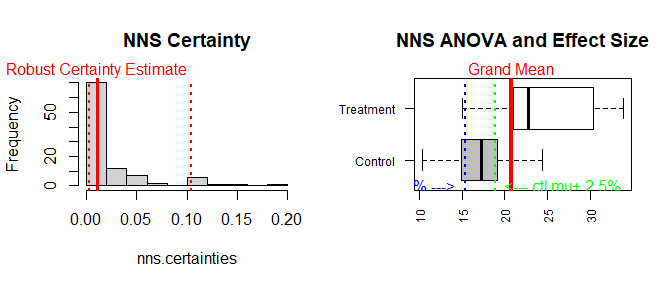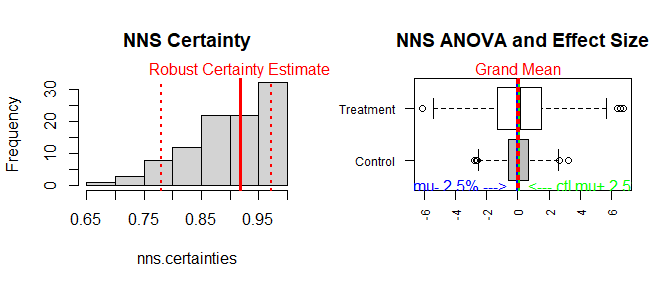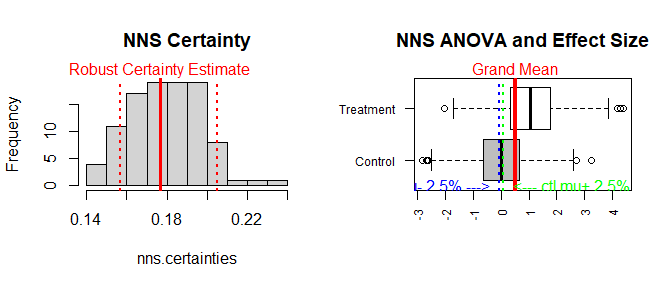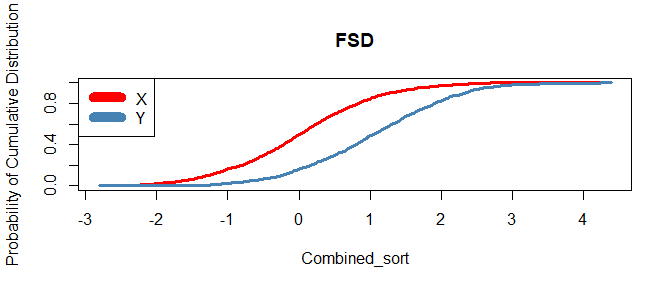# Getting Started with NNS: Comparing Distributions

library(NNS)
library(data.table)
require(knitr)
require(rgl)
require(meboot)

# Comparing Distributions

NNS offers a multitude of ways to test if distributions came from the same population, or if they share the same mean. The underlying function for these tests is NNS.ANOVA().

The output from NNS.ANOVA() is a Certainty statistic, which compares CDFs of distributions from several shared quantiles and normalizes the similarity of these points to be within the interval $$[0,1]$$, with 1 representing identical distributions. For a complete analysis of Certainty to common p-values and the role of power, please see the References.

## Test if Same Population

Below we run the analysis to whether automatic transmissions and manual transmissions have significantly different mpg distributions per the mtcars dataset.

The plot on the left shows the robust Certainty estimate, reflecting the distribution of Certainty estimates over 100 random permutations of both variables. The plot on the right illustrates the control and treatment variables, along with the grand mean among variables, and the confidence interval associated with the control mean.

mpg_auto_trans = mtcars[mtcars$am==1, "mpg"] mpg_man_trans = mtcars[mtcars$am==0, "mpg"]

NNS.ANOVA(control = mpg_man_trans, treatment = mpg_auto_trans, robust = TRUE)## $Control Mean ##  17.14737 ## ##$Treatment Mean
##  24.39231
##
## $Grand Mean ##  20.76984 ## ##$Control CDF
##  0.9152794
##
## $Treatment CDF ##  0.1670107 ## ##$Certainty
##  0.01009348
##
## $Lower Bound Effect ##  5.502308 ## ##$Upper Bound Effect
##  9.035466
##
## $Robust Certainty Estimate ##  0.0108829 ## ##$Lower 95% CI
##  0.002574409
##
## $Upper 95% CI ##  0.104652 The Certainty shows that these two distributions clearly do not come from the same population. This is verified with the Mann-Whitney-Wilcoxon test, which also does not assume a normality to the underlying data as a nonparametric test of identical distributions. wilcox.test(mpg ~ am, data=mtcars)  ## ## Wilcoxon rank sum test with continuity correction ## ## data: mpg by am ## W = 42, p-value = 0.001871 ## alternative hypothesis: true location shift is not equal to 0 ## Test if means are Equal Here we provide the output from NNS.ANOVA() and t.test() functions on two Normal distribution samples, where we are pretty certain these two means are equal. set.seed(123) x = rnorm(1000, mean = 0, sd = 1) y = rnorm(1000, mean = 0, sd = 2) NNS.ANOVA(control = x, treatment = y, means.only = TRUE, robust = TRUE, plot = TRUE)##$Control Mean
##  0.01612787
##
## $Treatment Mean ##  0.08493051 ## ##$Grand Mean
##  0.05052919
##
## $Control CDF ##  0.5218858 ## ##$Treatment CDF
##  0.4893919
##
## $Certainty ##  0.912545 ## ##$Lower Bound Effect
##  0.004184327
##
## $Upper Bound Effect ##  0.1277884 ## ##$Robust Certainty Estimate
##  0.9184417
##
## $Lower 95% CI ##  0.7799747 ## ##$Upper 95% CI
##  0.9712966
t.test(x,y)
##
##  Welch Two Sample t-test
##
## data:  x and y
## t = -0.96711, df = 1454.4, p-value = 0.3336
## alternative hypothesis: true difference in means is not equal to 0
## 95 percent confidence interval:
##  -0.20835512  0.07074984
## sample estimates:
##  mean of x  mean of y
## 0.01612787 0.08493051

## Test if means are Unequal

By altering the mean of the y variable, we can start to see the sensitivity of the results from the two methods, where both firmly reject the null hypothesis of identical means.

set.seed(123)
x = rnorm(1000, mean = 0, sd = 1)
y = rnorm(1000, mean = 1, sd = 1)

NNS.ANOVA(control = x, treatment = y,
means.only = TRUE, robust = TRUE, plot = TRUE)## $Control Mean ##  0.01612787 ## ##$Treatment Mean
##  1.042465
##
## $Grand Mean ##  0.5292966 ## ##$Control CDF
##  0.7862176
##
## $Treatment CDF ##  0.2197938 ## ##$Certainty
##  0.1824463
##
## $Lower Bound Effect ##  0.9617191 ## ##$Upper Bound Effect
##  1.085323
##
## $Robust Certainty Estimate ##  0.1769465 ## ##$Lower 95% CI
##  0.1569815
##
## \$Upper 95% CI
##  0.205275
t.test(x,y)
##
##  Welch Two Sample t-test
##
## data:  x and y
## t = -22.933, df = 1997.4, p-value < 2.2e-16
## alternative hypothesis: true difference in means is not equal to 0
## 95 percent confidence interval:
##  -1.1141064 -0.9385684
## sample estimates:
##  mean of x  mean of y
## 0.01612787 1.04246525

The effect size from NNS.ANOVA() is calculated from the confidence interval of the control mean and the specified y shift of 1 is within the provided lower and upper effect boundaries.

# Stochastic Dominance

Another method of comparing distributions involves a test for stochastic dominance. The first, second, and third degree stochastic dominance tests are available in NNS via:

• NNS.FSD()

• NNS.SSD()

• NNS.TSD()

NNS.FSD(x, y)##  "Y FSD X"

NNS.FSD() correctly identifies the shift in the y variable we specified when testing for unequal means.

## Stochastic Dominant Efficient Sets

NNS also offers the ability to isolate a set of variables that do not have any dominated constituents with the NNS.SD.efficient.set() function.

x2, x4, x6, x8 all dominate their preceding distributions yet do not dominate one another, and are thus included in the first degree stochastic dominance efficient set.

set.seed(123)
x1 = rnorm(1000)
x2 = x1 + 1
x3 = rnorm(1000)
x4 = x3 + 1
x5 = rnorm(1000)
x6 = x5 + 1
x7 = rnorm(1000)
x8 = x7 + 1

NNS.SD.efficient.set(cbind(x1, x2, x3, x4, x5, x6, x7, x8), degree = 1, status = FALSE)
 "x4" "x2" "x8" "x6"